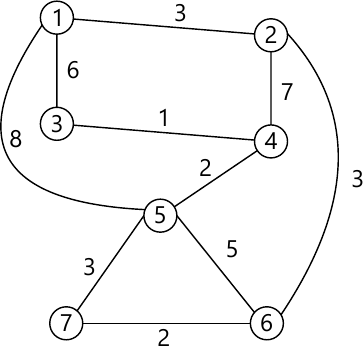MORE IN Data Structure & Algorithm Analysis
MU Information Technology (Semester 3)
Data Structure & Algorithm Analysis
December 2013
Total marks: --
Total time: --
INSTRUCTIONS
(1) Assume appropriate data and state your reasons
(2) Marks are given to the right of every question
(3) Draw neat diagrams wherever necessary

1 (a) What is Data structures and Abstract Data Type?
2 M
1 (b) What is AVL tree? Give example.
3 M
3 M
1 (d) What is Expression tree? Give example.
3 M
1 (e) What is Link List? State the different types of Link List.
3 M
1 (f) List out the properties of a symptotic notations.
3 M
1 (g) What is Data structures for Graph? Explain.
3 M

2 (a) What Doubly Linked List? Write an algorithm to implement following operations :-
(i) Insertion (All cases)
(ii) traversal (Forward and Backward)
10 M
2 (b) Define Binary search tree. Write an algorithm to implement Insertion and Deletion operation.
10 M

3 (a) Write a program to implement queue using array.
10 M
3 (b) Explain in brief insertion sort and shell sort.
10 M

4 (a) Explain in brief :-
(i) Directed Graph
(ii) Weighted Graph
(iii) Minimum spanning tree
10 M
4 (b) Find Minimum spanning tree for following graph using Prim's and krusal algorithm show various steps.10 M

5 (a) Write a program to convert INFIX expression into POSTFIX expression.
10 M
5 (b) Write a program to create singly Linked List and display the List.
10 M

6 (a) Write a program to implement a stack ADT using linked list.
10 M
6 (b) What is an AVL tree? Construct the AVL tree for following set of data. [Mention the type of rotation for each case].
1, 2, 3, 4, 8, 7, 6, 5, 11, 10, 12.
10 M

More question papers from Data Structure & Algorithm Analysis